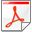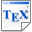[Bas14] N. Basset. Counting and generating permutations using timed languages. In Proc. Alfredo Viola (editor), LATIN 2014: Theoretical Informatics - 11th Latin American Symposium, Montevideo, Uruguay. Proceedings, volume 8392 of LNCS, pages 502-513, Springer. 2014. [pdf] [bib] Downloads:pdf (437 KB)bib Notes: The original publication is available at link.springer.com. Links: [Google] [Google Scholar] [CiteSeer] Abstract. The signature of a permutation sigma is a word whose letter of index i is d when sigma has a descent (i.e. sigma(i)> sigma(i+1) and is a when sigma has an ascent (i.e. sigma(i)< sigma(i+1). Combinatorics of permutations with a prescribed signature is quite well explored. Give a language over the alphabet {a,d}, we associate to it the class of permutations associated to a regular language. Here we state and address the two problems of counting and randomly generating for every classes of permutations associated to a regular language. First we give an algorithm that computes a closed form formula for the exponential generating function of such a class. Then we give an algorithm that given a regular language L and an integer n generates randomly the n-length permutations of the class associated to L in a uniform manner, i.e. all the permutations of length n with signature in L are equally probable to be returned. Both contributions are based on a geometric interpretation of a subclass of regular timed languages.